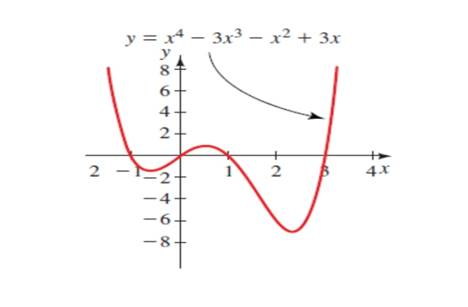# The equation y = x 4 − 3 x 3 − x 2 + 3 x ,and find the solution of the equation x 4 − 3 x 3 − x 2 + 3 x = 0 .### Precalculus: Mathematics for Calcu...

6th Edition
Stewart + 5 others
Publisher: Cengage Learning
ISBN: 9780840068071### Precalculus: Mathematics for Calcu...

6th Edition
Stewart + 5 others
Publisher: Cengage Learning
ISBN: 9780840068071

#### Solutions

Chapter 1.9, Problem 3E

a.

To determine

## To calculate: The equation y=x4−3x3−x2+3x ,and find the solution of the equation x4−3x3−x2+3x=0 .Expert Solution

The solution of the equation y=x43x3x2+3x is at which the curve touches the xaxis at 0 and 3.

### Explanation of Solution

Given information:

The equation y=x43x3x2+3x , gives the solution of the equation x43x3x2+3x=0 with the help of graph.

Formula used:

Steps to use this method to solve a quadratic polynomial ax4ax3ax2bx=0 .

Step 1. Check the equation for real numbers which satisfy the equation that means the value of x that makes the equation zero.

Step 2. Check the equation for x=1,0,1,2,3 .

Step 3. Now get the real roots of the equation.

Calculation:

The graph of the equation y=x43x3x2+3x :

So find the real roots of the equation y=x43x3x2+3x ,

Rewrite the equation x43x3x2+3x=0 :

Check for the real number x=1 in the equation x43x3x2+3x=0 .

x43x3x2+3x=0(1)43(1)3(1)2+3(1)=01+313=00=0

The value of x=1 satisfied the equation x43x3x2+3x=0 .

Now check for real number x=0 in the equation x43x3x2+3x=0 .

x43x3x2+3x=0(0)43(0)3(0)2+3(0)=00=0

The real no x=0 satisfied the equation x43x3x2+3x=0 .

Now check for real number x=1 in the equation x43x3x2+3x=0 .

x43x3x2+3x=0(1)43(1)3(1)2+3(1)=0131+3=00=0

The real no x=1 satisfied the equation x43x3x2+3x=0 .

Now check for real number x=2 in the equation x43x3x2+3x=0 .

x43x3x2+3x=0(2)43(2)3(2)2+3(2)=016244+6=06=0

The real number x=2 does not satisfied the equation.

Now check for real number x=3 in the equation x43x3x2+3x=0 .

x43x3x2+3x=0(3)43(3)3(3)2+3(3)=081819+9=00=0

The real no x=3 satisfied the equation x43x3x2+3x=0 .

Now we get the four real roots 1,0,1,3 .

The solution of the equation y=x43x3x2+3x is at which the curve touches the xaxis are 1,0,1,3 .

b.

To determine

### To calculate: The solution of the inequality x4−3x3−x2+3x≤0 .

Expert Solution

The solution of the inequality x43x3x2+3x0 is x(0,3) .

### Explanation of Solution

Given information:

The inequality x43x3x2+3x0 .

Formula used:

Steps to use this method to solve a quadratic polynomial ax4ax3ax2bx=0 .

Step 1. Check the equation for real numbers which satisfy the equation that means the value of x that makes the equation zero.

Step 2. Check the equation for x=1,0,1,2,3 .

Step 3. Now get the real roots of the equation.

Calculation:

The graph of the equation x43x3x2+3x0 :

So find the roots of the equation x43x3x2+3x0 ,

Rewrite the equation x43x3x2+3x=0 :

Check for the real number x=1 in the equation x43x3x2+3x=0 .

x43x3x2+3x=0(1)43(1)3(1)2+3(1)=01+313=00=0

The value of x=1 satisfied the equation x43x3x2+3x=0 .

Now check for real number x=0 in the equation x43x3x2+3x=0 .

x43x3x2+3x=0(0)43(0)3(0)2+3(0)=00=0

The real no x=0 satisfied the equation x43x3x2+3x=0 .

Now check for real number x=1 in the equation x43x3x2+3x=0 .

x43x3x2+3x=0(1)43(1)3(1)2+3(1)=0131+3=00=0

The real no x=1 satisfied the equation x43x3x2+3x=0 .

Now check for real number x=2 in the equation x43x3x2+3x=0 .

x43x3x2+3x=0(2)43(2)3(2)2+3(2)=016244+6=06=0

The real number x=2 does not satisfied the equation.

Now check for real number x=3 in the equation x43x3x2+3x=0 .

x43x3x2+3x=0(3)43(3)3(3)2+3(3)=081819+9=00=0

The real no x=3 satisfied the equation x43x3x2+3x=0 .

Now we get the four real roots 1,0,1,3 .

The solution of the equation y=x43x3x2+3x is at which the curve touches the xaxis are 1,0,1,3 .

The solution of the inequality consist of intervals [1,0][1,3] .

Thus, the solution of the inequality x43x3x2+3x0 is the area of curve below the x -axis is [1,0][1,3] .

### Have a homework question?

Subscribe to bartleby learn! Ask subject matter experts 30 homework questions each month. Plus, you’ll have access to millions of step-by-step textbook answers!